9-22.

Missing problem for Book CC3 => Chapter Ch9 => Lesson 9.1.2 => Problem 9-22

Created from orphaned homework help problem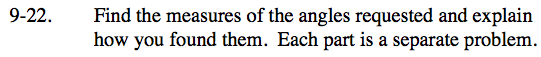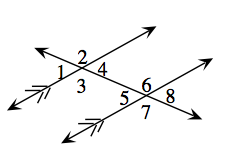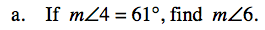Angle 4 is supplementary with angle 2, which corresponds to angle 6. Since the lines cut by the transversal are parallel, corresponding angles are equal.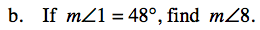Angle 1 and angle 4 are vertical angles, which are always equal. In addition, angle 4 and angle 8 are corresponding angles.

m∠1 = m∠4

m∠4 = m∠8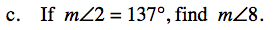Angle 2 and angle 6 are corresponding angles. Since the lines are parallel, they will have equal measure. Angle 6 and angle 8 are supplementary.